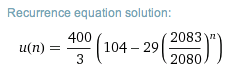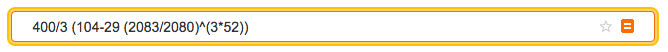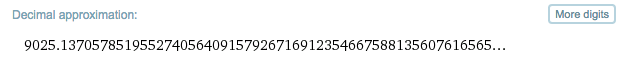﻿ Loans and Investments

#Loans and Investments

Need some practice with recursion and sequences first?

Loan and investment problems offer great applications of recursive sequences:

This section requires calculations that cannot reasonably be done by hand. Many calculators have the ability to work with recursive sequences. Or, there are forms on this page that you can use to do the calculations. Also, there are instructions on using WolframAlpha to work with recursive sequences.

## Paying Back a Loan

Suppose you are borrowing $\,\$22{,}000\,.$Interest is being charged at an annual rate of$\,5\%\,.$You plan to pay back$\,\$250\,$ each month; this payment goes to both interest and principal.

(a)
(b)   Write a recursive formula where $\,u_n\,$ gives the amount owed after $\,n\,$ months.
(c)
(d)  Find the total principal paid in the first year.
(e)  Find the total interest paid in the first year.
(f)  Putting it all together—the brief solution.

## Solution

### (a) Find the interest owed in the first month

• The annual interest rate is $\,5\% = 0.05\,.$
• The monthly interest rate is $\,\frac{0.05}{12}\,.$
• The interest owed in the first month is: $$(\22{,}000)(\frac{0.05}{12}) = \91.67$$

Notice that your monthly payment must be at least $\,\$91.67\,$to cover the interest. If you were to pay exactly$\,\$91.67\,$ the first month, then your debt remains $\,\$22{,}000\,.$If you were to pay$\,\$91.67\,$ each month for the next twenty years, you'd still owe $\,\$22{,}000\,$at the end of those twenty years. Obviously, you want to make sure you pay more than this each month, so the amount of debt decreases. You've decided to pay$\,\$250\,$ each month, so with this first payment you reduce your debt by $\,\$250 - \$91.67 = \$158.33\,.$That means you now owe$\,\$22{,}000 - \$158.33 = \$21{,}841.67\,,$ which will accrue a smidgeon less interest for the next month.

Look at the pattern for the amount you owe at the end of the first month. Dollar signs and commas are suppressed, to make it a bit easier on the eyes: \begin{align} &\cssId{s44}{\overset{\text{amt owed at beginning}}{\overbrace{\strut 22000}}}\cr\cr &\qquad \cssId{s45}{+ \overset{\text{interest accrued for the month}}{\overbrace{\strut (22000)(\frac{0.05}{12})}}}\cr\cr &\qquad \cssId{s46}{- \overset{\text{monthly payment}}{\overbrace{\strut \ \ 250\ \ }}}\cr\cr &\cssId{s47}{= \overset{\text{amount owed after one month}}{\overbrace{\strut 21841.67}}} \end{align}

Now we'll introduce the notation:

• Let $\,u_0\,$ denote the amount owed at time zero; that is, the initial debt.
• Let $\,u_1\,$ denote the amount owed after $\,1\,$ month (i.e., after $\,1\,$ payment).

Rewriting the formula above using this notation gives: \begin{align} &\cssId{s52}{\overset{\text{amt owed at beginning}}{\overbrace{\strut \ \ u_0\ \ }}}\cr\cr &\qquad \cssId{s53}{+ \overset{\text{interest accrued for the month}}{\overbrace{\strut (u_0)(\frac{0.05}{12})}}}\cr\cr &\qquad \cssId{s54}{- \overset{\text{monthly payment}}{\overbrace{\strut \ \ 250\ \ }}}\cr\cr &\cssId{s55}{= \overset{\text{amount owed after one month}}{\overbrace{\strut\ \ u_1\ \ }}} \end{align}

Rewrite the equation from right-to-left and factor, to get: $$\cssId{s57}{u_1 = (1 + \frac{0.05}{12})u_0 - 250}$$

### (b) Write a recursive formula where $\,u_n\,$ gives the amount owed after $\,n\,$ months

The pattern above gets applied month after month after month:

• Take the prior amount owed:  $\,u_{n-1}\,$
• Add in the interest accrued on this amount:  $\,u_{n-1}(\frac{0.05}{12})\,$
• Subtract off your payment:  $\,250\,$

Thus, the amount owed after $\,n\,$ months is: $$\cssId{s67}{\,u_n = (1 + \frac{0.05}{12})u_{n-1} - 250\,,\ \ \text{ for } n\ge 1}$$

### (c) Find the amount owed after one year of payback

Note:  For this part of the problem, you need a calculator that does recursion; or, you can use the JavaScript form below. Fill in the fields with the desired amounts. Then, click the button to compute $\,u_n\,.$ The initial (sample) values are from this current example.

The amount owed after one year (twelve months) is $\,u_{12}\,.$ From a calculator, or from the form below: $$\cssId{s77}{u_{12} = 20055.85}$$ Thus, you owe $\,\$20{,}055.85\,$after one year. The form below computes the amount owed after$\,n\,$payments on a loan payback with equal monthly payments: • You are borrowing$\,u_0\,$(in dollars). That is,$\,u_0\,$is the amount owed at time zero (the start of the loan). • Interest is being charged at an annual interest rate$\,i\%\,.$For example, if the interest rate is$\,5\%\,,$then$\,i = 5\,.$That is,$\,i\,$does not include the percent sign. • You are paying back$\,\$B\,$ each month.

For example, if you pay back $\,\$250\,,$then$\,B = 250\,.$That is,$\,B\,$does not include the dollar sign. •$\,u_n\,$is the amount owed (principal plus interest) after$\,n\,$payments: $$\begin{gather} u(n) = u_n = \bigl(1 + \frac{i/100}{12}\bigr)u_{n-1} - B\cr\cr \text{for}\ n = 1,2,3,\ldots \end{gather}$$ ## JavaScript Form: The amount owed after$\,n\,$payments on a loan payback with equal monthly payments$u_0 =\,$amount borrowed, in dollars: Do not include commas or dollar sign. Annual interest rate,$\,i\,,$as a percent: Do not include the percent sign. Amount paid back each month,$\,B\,,$in dollars: Do not include commas or dollar sign. Value of$\,n\,$for which you want$\,u_n\,$: By clicking the button below, you will calculate the amount still owed after$\,n\,$monthly payments. ### (d) Find the total principal paid in the first year The total principal paid in the first year is: $$\cssId{s109}{\22{,}000 - \20{,}055.85 = \1944.15}$$ ### (e) Find the total interest paid in the first year How much money did you actually send to the bank in the first year? Twelve payments of \$250 each: $$\cssId{s113}{(12)(\250) = \3000}$$ Of this, you know from part (d) that $\,\$1944.15\,went to principal—that is, reduced your debt. The remainder is interest—your payment for the privilege of using the bank's money for the year. Thus, the total interest paid in the first year is: $$\cssId{s117}{(12)(\250) - \1944.15 = \1055.85}$$ ### (f) Putting it all together—the brief solution Here's the brief solution: • (a) The interest owed in the first month is: $$\cssId{s121}{(\22{,}000)(\frac{0.05}{12}) = \91.67}$$ • (b) The recursive formula is: \begin{align} &\cssId{s123}{u_0 = 22000}\cr &\cssId{s124}{u_n = (1 + \frac{0.05}{12})u_{n-1} - 250\,,\ \ \text{ for } n\ge 1} \end{align} • (c) From the calculator or form: $$\cssId{s126}{u_{12} = \20{,}055.85}$$ • (d) The total principal paid in the first year is: $$\cssId{s128}{\22{,}000 - \20{,}055.85 = \1944.15}$$ • (e) The total interest paid in the first year is: $$\cssId{s130}{(12)(\250) - \1944.15 = \1055.85}$$ ### Read-Through, Part 2 ## Saving for the Future You are saving for the future. Your initial deposit is\,\$4100\,.$ Interest is being earned at an annual rate of $\,5\%\,,$ compounded monthly. You will contribute an additional $\,\$120\,$each month. (a) (b) Write a recursive formula where$\,u_n\,$gives the amount saved (principal plus interest) after$\,n\,$months. (c) Then, find the amount saved (principal plus interest) after$\,7\,$years. (d) Find the total amount of money you contributed (principal only) during these$\,7\,$years. (e) Find the total interest earned during these$\,7\,$years. ## Solution ### (a) Find the interest earned in the first month The interest earned in the first month is: $$\cssId{sb18}{(\4{,}100)(\frac{0.05}{12}) = \17.08}$$ ### (b) Write a recursive formula where$\,u_n\,$gives the amount saved (principal plus interest) after$\,n\,months The recursive formula is: \begin{align} &\cssId{sb21}{u_0 = 4100}\cr &\cssId{sb22}{u_n = (1 + \frac{0.05}{12})u_{n-1} + 120\,,\ \ \text{ for } n \ge 1} \end{align} ### (c) Find the amount saved (principal plus interest) after\,7\,$years Note that$\,7\,$years is$\,7(12) = 84\,$months. From the calculator, or from the form below: $$\cssId{sb26}{u_{84} = 17853.39}$$ Thus, you have saved (principal plus interest)$\,\$17{,}853.39\,$ after $\,7\,$ years.

The form below computes the amount saved (principal plus interest) after $\,n\,$ equal monthly payments:

• Your initial deposit is $\,u_0\,$ (in dollars).

That is, $\,u_0\,$ is the amount saved at time zero (the start of your savings program).

• Interest is being earned at an annual rate of $\,i\%\,,$ compounded monthly.

For example, if the interest rate is $\,5\%\,$, then $\,i = 5\,.$ That is, $\,i\,$ does not include the percent sign.

• You are contributing an additional $\,\$C\,$each month. For example, if you contribute$\,\$120\,,$ then $\,C = 120\,.$ That is, $\,C\,$ does not include the dollar sign.

• $\,u_n\,$ is the amount saved (principal plus interest) after $\,n\,$ monthly contributions: \begin{align} \cssId{sb38}{u(n)}\cr &\cssId{sb39}{= u_n}\cr &\cssId{sb40}{= \bigl(1 + \frac{i/100}{12}\bigr)u_{n-1} + C \text{ for }\ n = 1,2,3,\ldots} \end{align}

## JavaScript Form: The amount saved after $\,n\,$ equal monthly payments

$u_0 =\,$ initial deposit, in dollars:
Do not include commas or dollar sign.

Annual interest rate, $\,i\,,$ as a percent:
Do not include the percent sign.

Amount contributed each month, $\,C\,,$ in dollars:
Do not include commas or dollar sign.

Value of $\,n\,$ for which you want $\,u_n\,$:
By clicking the button below, you will compute the amount saved (principal plus interest) after $\,n\,$ monthly contributions.
You can use an asterisk, ‘*’, to denote multiplication.

### (d) Find the total amount of money you contributed (principal only) during these $\,7\,$ years

The total amount of money you contributed (principal only) during these $\,7\,$ years is: $$\cssId{sb59}{\4100 + 7(12)(\120) = \14{,}180.00}$$

### (e) Find the total interest earned during these $\,7\,$ years

The total interest earned during these $\,7\,$ years is: $$\cssId{sb62}{\17{,}853.39 - \14{,}180.00 = \3,673.39}$$

## Using WolframAlpha to Work with Recursive Sequences

Find $\,u_n\,$ for $\,n = 3\cdot 52\,$, if: \begin{align} &\cssId{sb65}{u_0 = 10,000}\cr &\cssId{sb66}{u_n = (1 + \frac{0.075}{52})u_{n-1} - 20\,,\ \ \text{ for }\ n \ge 1} \end{align}

• ### Step 1

Put the information into WolframAlpha:

u(0) = 10000, u(n) = (1 + 0.075/52)*u(n-1) - 20

• ### Step 2As part of your answer, you get this recurrence equation solution, which is a nonrecursive description of the sequence:• ### Step 3

If you click on the recurrence equation solution, then WolframAlpha puts it in the input box for you. This is much safer than typing it in yourself!• ### Step 4

Change the value of $\,n\,$ to the desired value. If the value of $\,n\,$ involves computations, then (to ensure correct order of operations) put it inside parentheses, as shown here:• ### Step 5

Scroll down to the decimal approximation:So, if you borrow $\,\$10,000\,$at a$\,7.5\%\,$annual interest rate and pay back$\,\$20\,$ per week, then after $\,3\,$ years you will still owe $\,\$9025.14\,.\$